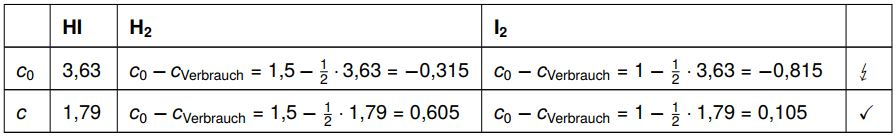# Where can we use chemistry

## Law of mass action

We summarize and solve for the size we are looking for, in this case x:
\ begin {align *}
\ begin {array} {crcll}
% & 51 & = & \ frac {x ^ 2} {1 {,} 5 - 0 {,} 5 x - 0 {,} 75 x + 0 {,} 25x ^ 2} & \
& 51 & = & \ frac {x ^ 2} {0 {,} 25x ^ 2 - 1 {,} 25 x + 1 {,} 5} & | \ cdot (0 {,} 25x ^ 2 - 1 {, } 25 x + 1 {,} 5) \
\ Leftrightarrow & 51 \ cdot (0 {,} 25x ^ 2 - 1 {,} 25 x + 1 {,} 5) & = & x ^ 2 & \
\ Leftrightarrow & 12 {,} 75x ^ 2 - 63 {,} 75 x + 76 {,} 5 & = & x ^ 2 & | -x ^ 2 \
\ Leftrightarrow & 11 {,} 75x ^ 2 - 63 {,} 75 x + 76 {,} 5 & = & 0 &
\ end {array}
\ end {align *}
There is now a quadratic equation in normal form, which we solve with the midnight formula (pq formula is also possible, but for this the equation must first be divided by 11.75):
\ begin {align *}
x_ {1,2} = \ frac {63 {,} 75 \ pm \ sqrt {63 {,} 75 ^ 2 - 4 \ times 11 {,} 75 \ times 76 {,} 5}} {2 \ times 11 {,} 75} \ Rightarrow x_1 = 3 {,} 63 \ wedge x_2 = \ underline {\ underline {1 {,} 79}}
\ end {align *}
For the hydrogen iodide concentration that arises and is present in equilibrium, we previously defined the variable x. We put the possible values ​​for x in the table and quickly see which value is the correct one.The first solution would result in negative concentrations for hydrogen and iodine when equilibrium was established. Since concentration can never be negative, this result is wrong. The second solution gives us positive values ​​for this. So only the second solution can be correct.

So we now know all the concentrations we are looking for:
\ begin {align *}
c_ \ text {GG} ({HI}) = 1 {,} 79 \ \ frac {{mol}} {{L}}, \ quad c_ \ text {GG} ({H_2}) = 0 {,} 605 \ \ frac {{mol}} {{L}}, \ quad c_ \ text {GG} ({I_2}) = 0 {,} 105 \ \ frac {{mol}} {{L}}
\ end {align *}